Question

A hypothetical planet has a mass one-third of and a radius three times that of Earth. What is the acceleration due to gravity on the planet in terms of g, the acceleration due to gravity on Earth?

1.phucdien

Gravity of hypothetical planet is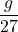.

Explanation:

Let the mass of Earth be ‘M’ and radius be ‘R’.

Given:

Mass of the hypothetical planet (m) = one-third of Earth’s mass =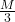Radius of hypothetical planet (r) = 3 times Earth’s radius =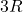We know that, acceleration due to gravity of a planet of mass ‘M’ and radius ‘R’ is given as: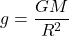Now, the above is the value of ‘g’ for Earth.

Now, acceleration due to gravity of hypothetical planet is given as: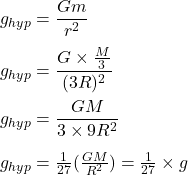So, the hypothetical planet is 1/27 times of the gravity of the Earth.